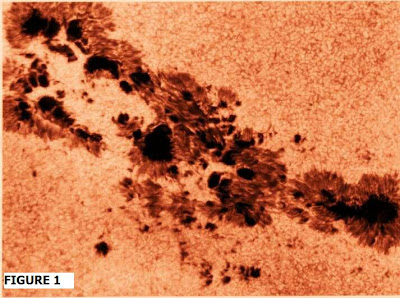## Sunday, August 22, 2010

### The Hall E-Field: Can it work in large sunspots?At the American Astronomical Society Solar Physics Division Meeting last July, my paper focused on whether the Hall electric (E-)field and electrodynamics was feasible for a large sunspot group (see Fig. 1). I showed that, at least for large sunspots within the group, it can work.

The Hall electric field E (H) , defined generically (Bray and Loughhead, 1964) , is:
E (H) = 1/nec [j x B/u]
= (j B/ necu) = j/ oH

where n is the particle density, e the electronic charge, c the speed of light, u is the magnetic permeability and o, the conductivity, H is magnetic intensity (H = B/u) and E(H) is typically ignored in solar flare applications. However, Sen and White (1972, Solar Phys., 23, 146.) were among the first to show a possible role for it in conjunction with the two-stream (Farley) instability and the triggering of a solar flare using a “dynamo” model. Their motivation was to see if a generic Hall field could provide a maximal flare energy equal or greater than 10 ^32 ergs (10 ^25 J) using reasonable assumptions for the flare volume and duration. They argued the flare trigger manifests when the electron drift velocity (v_d) associated with the Hall current density ( J(H)) exceeds the ion thermal velocity (V_ith). Sen and White quantified the critical drift velocity threshold value as: v(d) ~ 1 km/s.

What I found is the particular model of Sen and White didn't really work for a large flare, or large spot group, and moreover, a number of errors in their energy computations showed the energy available was not nearly as high as they claimed. My own version of the sunspot model is depicted in Figure 2.

The electric field, E, depicted in Fig. 2, arises from the designated directions of the vectors (v, B) and application of the "three-fingered rule" (familiar to all students of college general physics), yielding the effective Hall E-Field, E(H). It is interesting that its direction is opposite the direction of E(o): the “dynamo electric field” proposed by Kan at al (Solar phys., 1983, Fig. 1 therein) for their dynamo flare model. The Hall E-field, with vector E(H) directed inward, is computed using velocity V(theta), and modifying Sen and White’s (1972) quantitative form.

The resulting computation yields: E(H) = » 6.3 x 10 ^-3 statvolt/cm

for the particular large sunspot examined in the solar active region designated AR2776.

In the Sen & White model, the Hall field (and current density) was deduced by assuming a longitudinal field H( z) at the photosphere, and velocity field defined by V(r) synonymous with convective motion outwards from a spot umbra. In my own Hall-field model, the transverse induction field component at the photosphere was used (B(t)) which for the combined loop-arch field translates into the poloidal component (B (p) ).

Instead of a velocity field radially directed outwards (from umbra or presumptive solar loop "footpoint" center) the velocity is rotary (e.g. foot points rotate about axis z) with v = vn + r w l, where w is the angular velocity.

Thus, for the magnitude of the E-field, if E = v X B(t).

[E] = V (p) B

Where V(p) is the poloidal velocity. Note: the Hall term results from using:

v = [ m_e v_e + m_i v_i ] / (m_e + m_i )

for the center of mass flow velocity, instead of the electron velocity v_e in the motional term.

One of the other major advantages of my model, is that it can be generalized for a quadripolar sunspot model (which the attached image is more likely to embody). In this case there could reasonably be four footpoints in relative motion with respect to one another. In this case, there could be some kind of intrinsic dynamo which might feasibly operate. I will look at this in much more detail in a future blog entry.

 Bray, R.J. and Loughhead, R.E.: 1964, Sunspots, Dover Publications, p. 269.